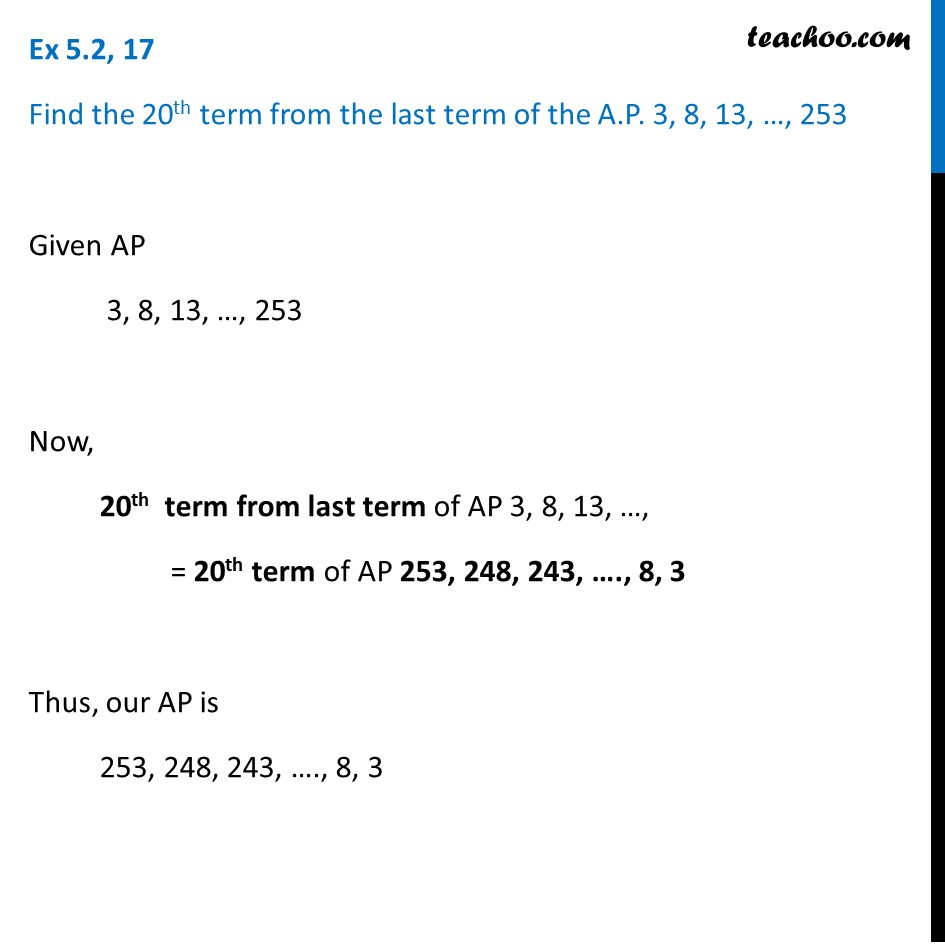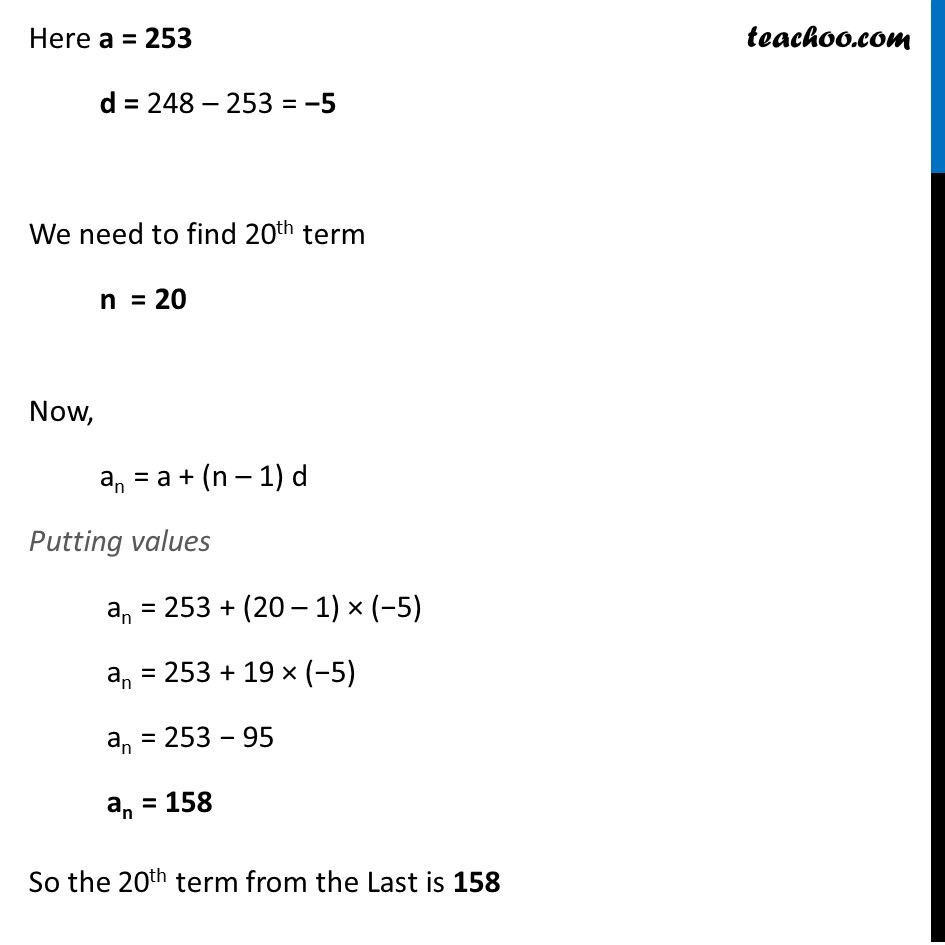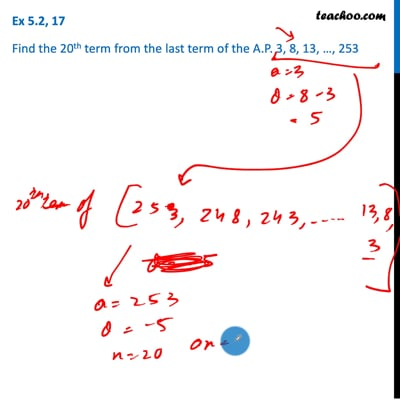Finding nth term from end

Chapter 5 Class 10 Arithmetic Progressions
Concept wiseThis video is only available for Teachoo black users

Introducing your new favourite teacher - Teachoo Black, at only ₹83 per month

### Transcript

Ex 5.2, 17 Find the 20th term from the last term of the A.P. 3, 8, 13, …, 253 Given AP 3, 8, 13, …, 253 Now, 20th term from last term of AP 3, 8, 13, …, = 20th term of AP 253, 248, 243, …., 8, 3 Thus, our AP is 253, 248, 243, …., 8, 3 Here a = 253 d = 248 – 253 = −5 We need to find 20th term n = 20 Now, an = a + (n – 1) d Putting values an = 253 + (20 – 1) × (−5) an = 253 + 19 × (−5) an = 253 − 95 an = 158 So the 20th term from the Last is 158